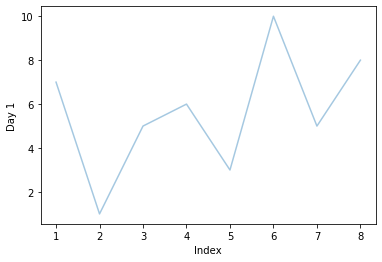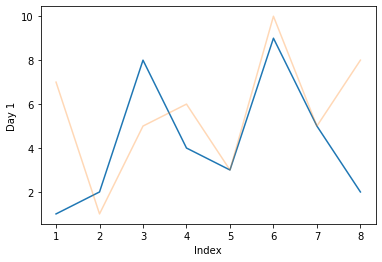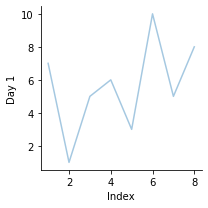# Transparency of Seaborn Plots

Manav Narula Oct 12, 2021 May 22, 2021

In this tutorial, we will discuss how to control the transparency of seaborn plots in Python.

To control the transparency of plots, we can use the `alpha` argument within the plot function. By default, its value is 1. The value of this parameter ranges from 0 to 1, with the plot getting more transparent and invisible as the value reaches 0. It can be used directly in most of the plot functions of the seaborn module.

For example,

``````import pandas as pd
import matplotlib.pyplot as plt
import seaborn as sns

df = pd.DataFrame({"Day 1": [7,1,5,6,3,10,5,8],
"Index": [1,2,3,4,5,6,7,8],
})

sns.lineplot(data =df, y = 'Day 1', x= 'Index', alpha  = 0.4)
``````Note that the value of the `alpha` parameter can be more than 1. However, the farther its value goes from an integer, the more transparent the plot gets. Similarly, the closer it gets to the integer, the brighter the plot gets. Given that this can be a little complicated to understand, keeping the parameter between 0 and 1 only.

In the above example, we created a line plot using the seaborn module and made the plot line more transparent by setting the `alpha` parameter as 0.4.

On one plot, we can specify the `alpha` parameter once only. But, transparency can be used as a way to differentiate different variables.

For example,

``````import pandas as pd
import matplotlib.pyplot as plt
import seaborn as sns

df = pd.DataFrame({"Day 1": [7,1,5,6,3,10,5,8],
"Day 2" : [1,2,8,4,3,9,5,2],
"Index": [1,2,3,4,5,6,7,8]})
sns.lineplot(data =df, x = 'Index', y = 'Day 2', alpha  = 1)
sns.lineplot(data =df, x = 'Index', y = 'Day 1', alpha  = 0.3)
``````In the above code, we plotted two variables on the same graph. Basically, one plot is plotted over the other plot, and both have different values for `alpha`. We can differentiate between the two values based on the transparency since one variable is made more transparent than the other.

The `alpha` argument can be used in different FacetGrid, PairGrid objects also. For such objects, we can use the parameter in the `map()` function also.

See the following code.

``````import pandas as pd
import matplotlib.pyplot as plt
import seaborn as sns

df = pd.DataFrame({"Day 1": [7,1,5,6,3,10,5,8],
"Index": [1,2,3,4,5,6,7,8],
})
g = sns.FacetGrid(df)
g.map(sns.lineplot, 'Index', 'Day 1', alpha = 0.4)
``````Author: Manav Narula

Manav is a IT Professional who has a lot of experience as a core developer in many live projects. He is an avid learner who enjoys learning new things and sharing his findings whenever possible.